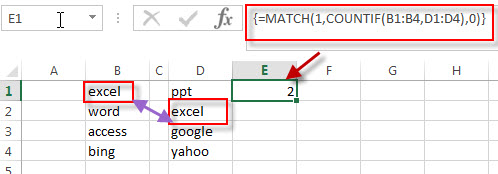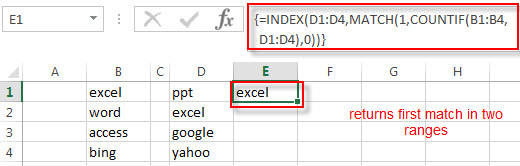# How to Get the First Match in Two Excel Ranges

This post will guide you how to get the first match value in two ranges in excel. How to return the first occurrence of the value in two excel ranges.

## Get the First Match in two Ranges

If you want to find the first match between two excel ranges, you can use a combination of the INDEX function, the MATCH function and COUNTIF function to create a new formula. For example, to get the first occurrence of the value between range B1:B4 and D1:D4, then extract the first value from range D1:D4, you can write down the following excel formula:

`=INDEX(D1:D4,MATCH(1,COUNTIF(B1:B4,D1:D4),0))`

Note: when you enter the above array formula, you must hold the Control and Shift keys down, then press Enter. Then the formula bar should add curly braces surrounding the array formula.

Let’s see how this formula works:

= COUNTIF(B1:B4,D1:D4)

The COUNTIF function will count the number of the value in the range D1:D4 cells that equal to the values in the range B1:B4. It will compare each values in range D1:D4 if it appear in range B1:B4. It returns an array result like this:

`={0;1;0;0}`

= MATCH(1,COUNTIF(B1:B4,D1:D4),0)The MATCH function returns the position of the first occurrence of the lookup value “1” in array results that returned by the COUNTIF function. It returns 2. And this value is also the position of the first match in those two ranges. And then it goes into INDEX function as its row_num.

=INDEX(D1:D4,MATCH(1,COUNTIF(B1:B4,D1:D4),0))The INDEX function returns a value from range D1:D4 based on the position that returned by the MATCH function. It returns “excel”.

### Related Formulas

• Find the nth Smallest Value
You can use the SMALL function to get the 1st, 2nd, 3rd, or nth smallest value in an array or range. Also you can use the SMALL function within the INDEX function to extract the relative value of the same row…
• Extract a List of Unique Values from a Column Range
f you want to extract a list of unique items from a column or range, you can use a combination of the IFERROR function, the INDEX function, the MATCH function and the COUNTIF function to create an array formula…..
• Get Cell Address of a Lookup Value
If you want to lookup a value in a range or column and return the cell address of the first match of lookup value, you can use a combination with the CELL function, the INDEX function and the MATCH function…

### Related Functions

• Excel COUNTIF function
The Excel COUNTIF function will count the number of cells in a range that meet a given criteria. This function can be used to count the different kinds of cells with number, date, text values, blank, non-blanks, or containing specific characters.etc.= COUNTIF (range, criteria)…
• Excel INDEX function
The Excel INDEX function returns a value from a table based on the index (row number and column number)The INDEX function is a build-in function in Microsoft Excel and it is categorized as a Lookup and Reference Function.The syntax of the INDEX function is as below:= INDEX (array, row_num,[column_num])…
• Excel MATCH function
The Excel MATCH function search a value in an array and returns the position of that item.The syntax of the MATCH function is as below:= MATCH  (lookup_value, lookup_array, [match_type])….